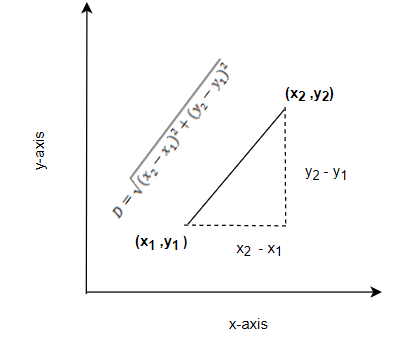# Distance between two points CalculatorNote
• Enter the values of Coordinates of the two points
• Click on the calculate button.

Formula And concept used
$D = \sqrt {(x_2 - x_1)^2 + (y_2 - y_1)^2}$

### Distance between two points Calculator

Example of Few questions where you can use this Distance between two points Calculator
1. Find the Centroid of a Triangle.
i. A(2,3), B(4 ,5)
ii. A(-1,3), C(2,2)
iii. B(2 ,5) , C(1,7)
iv. A(1,1), C(9,5)